# Concept 1 Linear Equations What are Linear Equations

• Slides: 33Concept 1 Linear EquationsWhat are Linear Equations?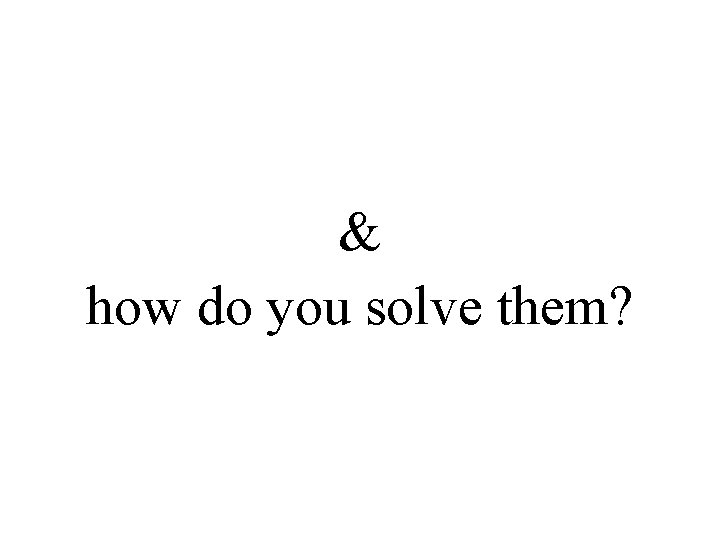& how do you solve them?Solving Linear Equations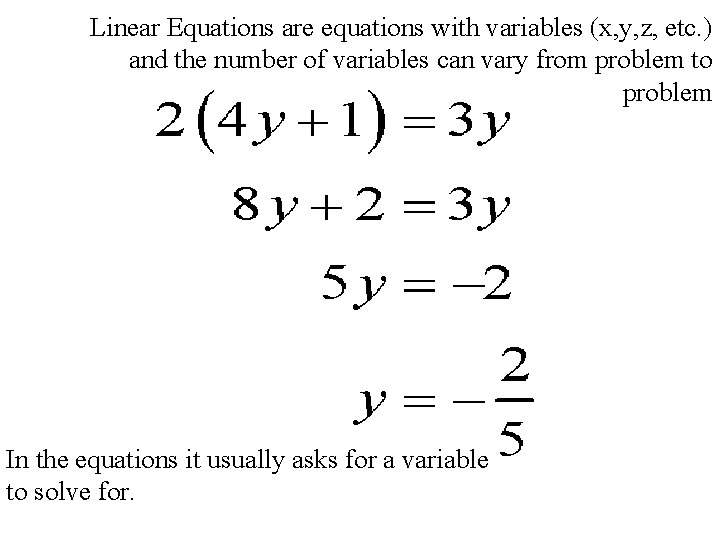Linear Equations are equations with variables (x, y, z, etc. ) and the number of variables can vary from problem to problem In the equations it usually asks for a variable to solve for.Identifying what is what…In the linear equation there are different things to identify first what are you solving for, if it is a two variable equation, or what are coefficients and how do they work and the process of solving the linear equations.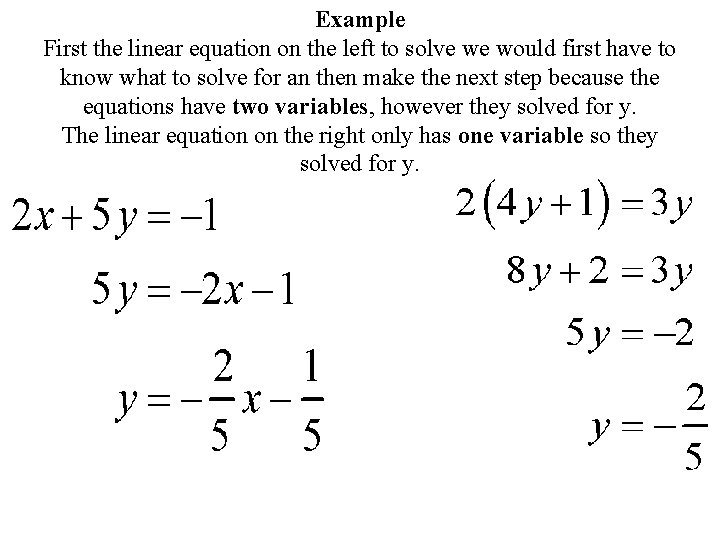Example First the linear equation on the left to solve we would first have to know what to solve for an then make the next step because the equations have two variables, however they solved for y. The linear equation on the right only has one variable so they solved for y.Here we have the perfect example of coefficients. If you look at 4 y and 3 y, these are variables that have coefficients attached to them. Numbers that come with variables are called COEFFICIENTSThe Process Of Solving…. .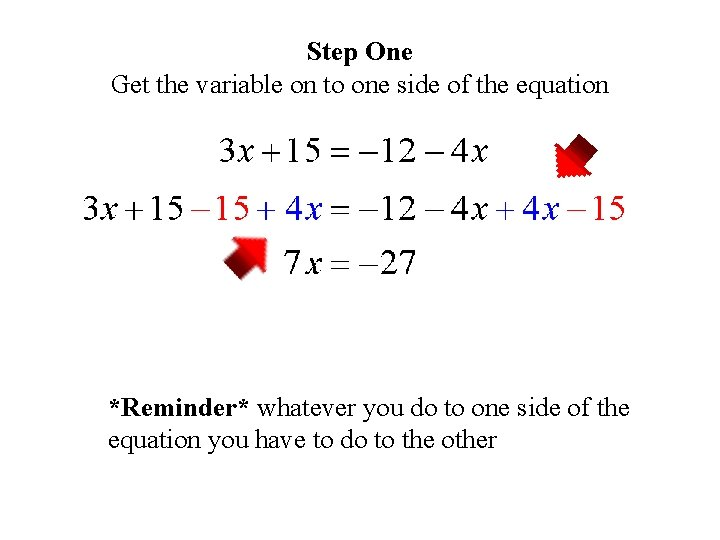Step One Get the variable on to one side of the equation *Reminder* whatever you do to one side of the equation you have to do to the other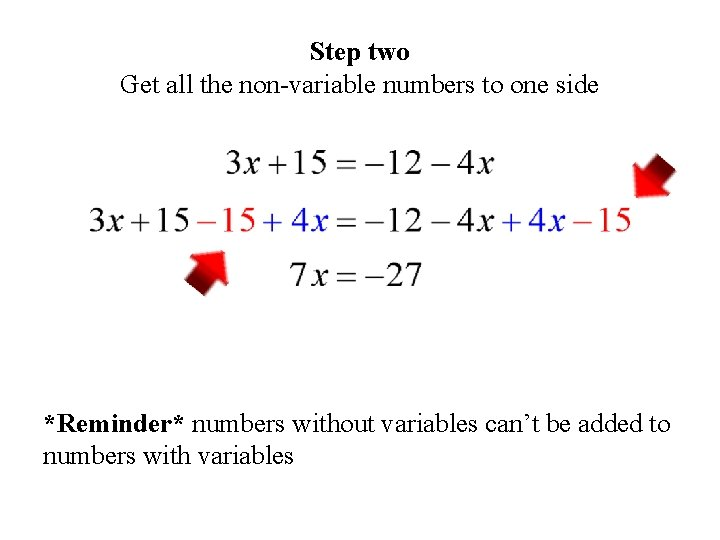Step two Get all the non-variable numbers to one side *Reminder* numbers without variables can’t be added to numbers with variables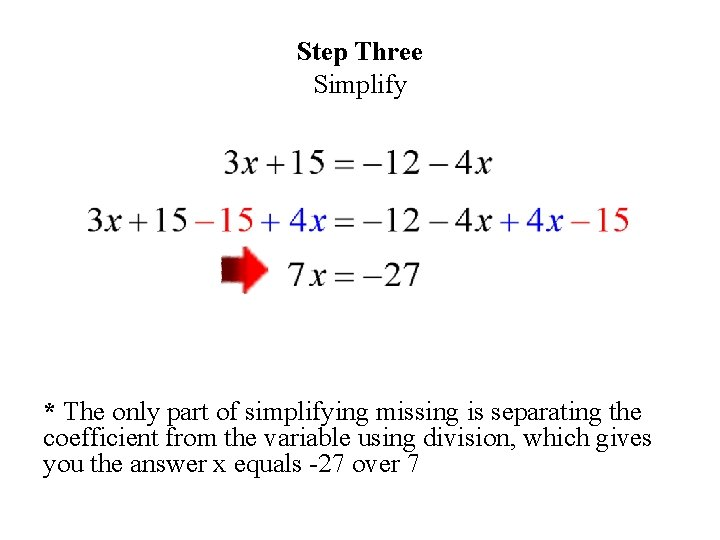Step Three Simplify * The only part of simplifying missing is separating the coefficient from the variable using division, which gives you the answer x equals -27 over 7Quick Review • Remember what you do to one side of the equation you must do to the other. • Remember to combine like-terms • Solve for what the problem is asking you forHow to Graph Linear EquationsLinear Equations are equations with variables and when placed (plotted) onto a graph they create a linear line.And to graph them you have to place them into a formula known as slope intercept form.But Wait……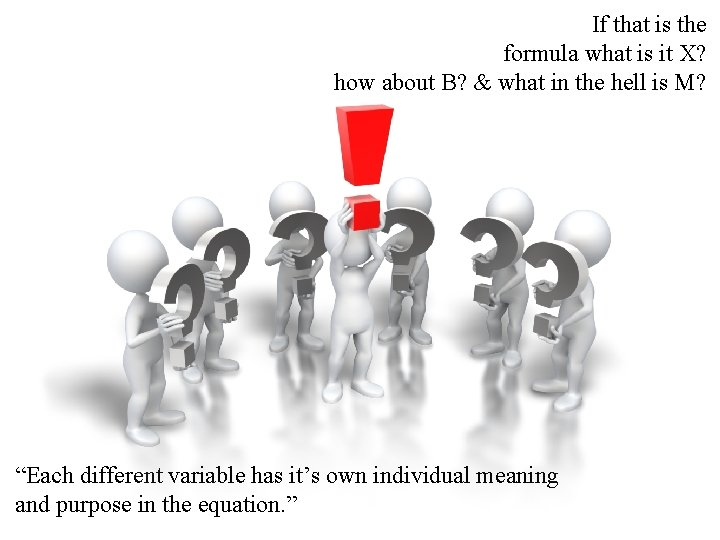If that is the formula what is it X? how about B? & what in the hell is M? “Each different variable has it’s own individual meaning and purpose in the equation. ”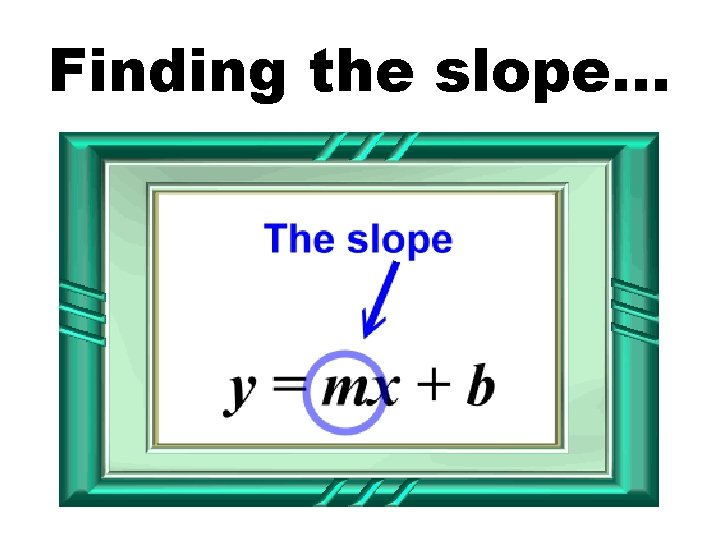Finding the slope…Slope controls the rate of change at which the linear equation moves along the graph when plotting points The slope (M) is always in front of the X in the equationAnother way to look at slope is to identify it as rise over run. Which is often written in the form of a faction Rise = movement on y-axis Run = movement on x-axis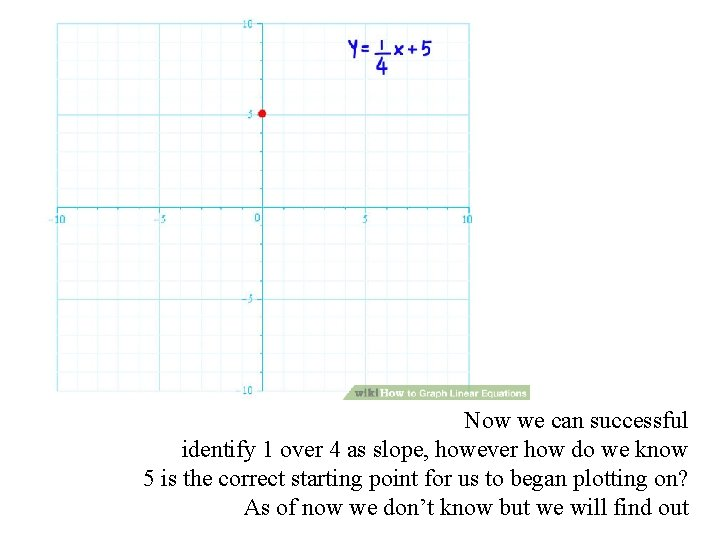Now we can successful identify 1 over 4 as slope, however how do we know 5 is the correct starting point for us to began plotting on? As of now we don’t know but we will find outB = Y-intercept…Y- Intercept (B) controls the starting point for the Linear Equations when graphing, much like slope (M) controls the rate of change Y-Intercept (B) is usually located at the end of the equation, but its most identifiable because it doesn’t have any variable with it just a solo number.But Wait There’s MORE!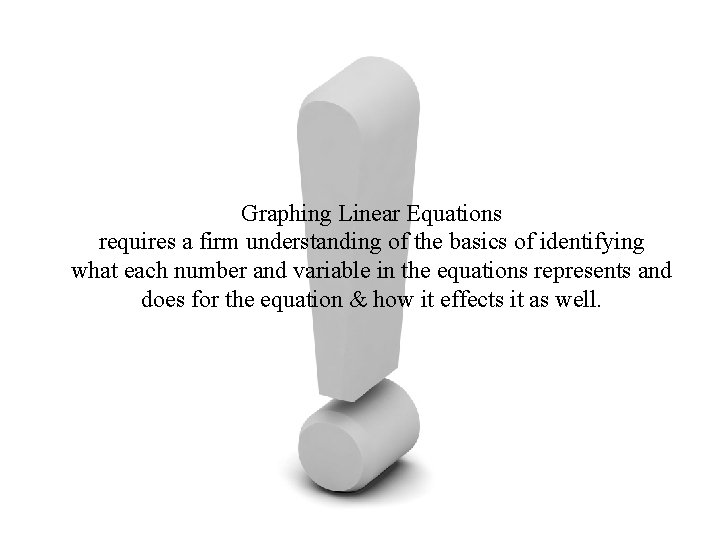Graphing Linear Equations requires a firm understanding of the basics of identifying what each number and variable in the equations represents and does for the equation & how it effects it as well.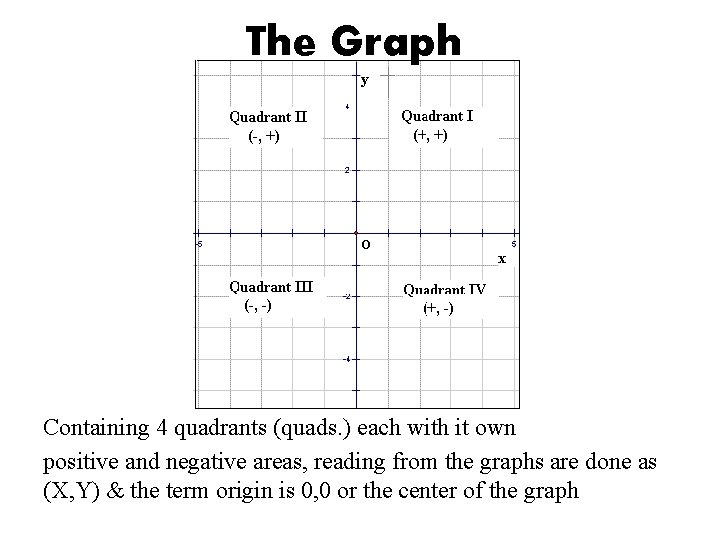The Graph Containing 4 quadrants (quads. ) each with it own positive and negative areas, reading from the graphs are done as (X, Y) & the term origin is 0, 0 or the center of the graphPutting it ALL TOGETHER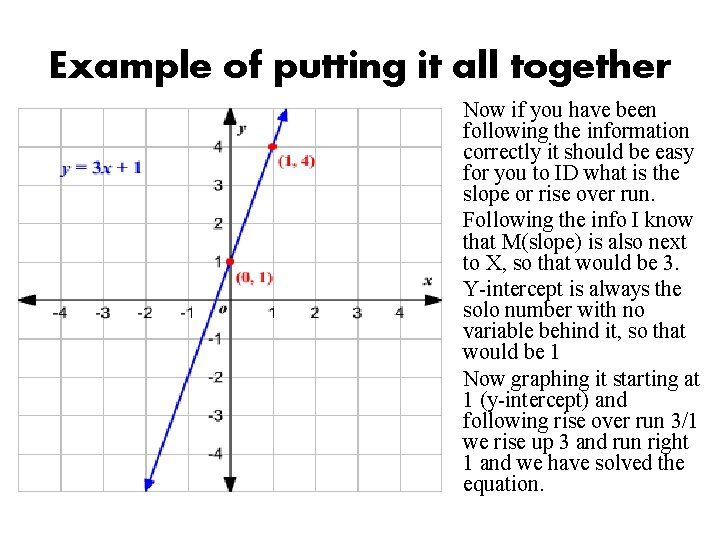Example of putting it all together Now if you have been following the information correctly it should be easy for you to ID what is the slope or rise over run. Following the info I know that M(slope) is also next to X, so that would be 3. Y-intercept is always the solo number with no variable behind it, so that would be 1 Now graphing it starting at 1 (y-intercept) and following rise over run 3/1 we rise up 3 and run right 1 and we have solved the equation.Quick Review • Slope intercept form is needed first to graph the linear equation • Followed by identifying the slope and the Yintercept • To graph you use the Y-intercept to plot the first point on the graph, then use the slope (M) and rise over run to plot the next point.